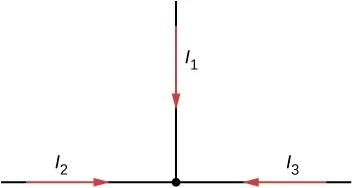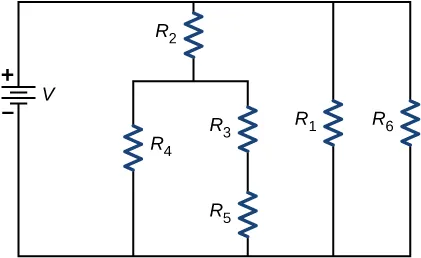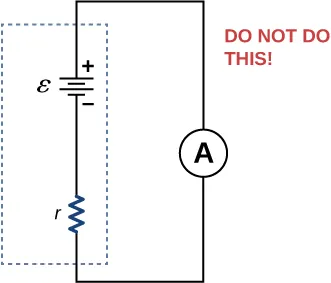University Physics Volume 2

# Conceptual Questions

University Physics Volume 2Conceptual Questions

### 10.1Electromotive Force

1.

What effect will the internal resistance of a rechargeable battery have on the energy being used to recharge the battery?

2.

A battery with an internal resistance of r and an emf of 10.00 V is connected to a load resistor $R=rR=r$. As the battery ages, the internal resistance triples. How much is the current through the load resistor reduced?

3.

Show that the power dissipated by the load resistor is maximum when the resistance of the load resistor is equal to the internal resistance of the battery.

### 10.2Resistors in Series and Parallel

4.

A voltage occurs across an open switch. What is the power dissipated by the open switch?

5.

The severity of a shock depends on the magnitude of the current through your body. Would you prefer to be in series or in parallel with a resistance, such as the heating element of a toaster, if you were shocked by it? Explain.

6.

Suppose you are doing a physics lab that asks you to put a resistor into a circuit, but all the resistors supplied have a larger resistance than the requested value. How would you connect the available resistances to attempt to get the smaller value asked for?

7.

Some light bulbs have three power settings (not including zero), obtained from multiple filaments that are individually switched and wired in parallel. What is the minimum number of filaments needed for three power settings?

### 10.3Kirchhoff's Rules

8.

Can all of the currents going into the junction shown below be positive? Explain.9.

Consider the circuit shown below. Does the analysis of the circuit require Kirchhoff’s method, or can it be redrawn to simplify the circuit? If it is a circuit of series and parallel connections, what is the equivalent resistance?10.

Do batteries in a circuit always supply power to a circuit, or can they absorb power in a circuit? Give an example.

11.

What are the advantages and disadvantages of connecting batteries in series? In parallel?

12.

Semi-tractor trucks use four large 12-V batteries. The starter system requires 24 V, while normal operation of the truck’s other electrical components utilizes 12 V. How could the four batteries be connected to produce 24 V? To produce 12 V? Why is 24 V better than 12 V for starting the truck’s engine (a very heavy load)?

### 10.4Electrical Measuring Instruments

13.

What would happen if you placed a voltmeter in series with a component to be tested?

14.

What is the basic operation of an ohmmeter as it measures a resistor?

15.

Why should you not connect an ammeter directly across a voltage source as shown below?### 10.5RC Circuits

16.

A battery, switch, capacitor, and lamp are connected in series. Describe what happens to the lamp when the switch is closed.

17.

When making an ECG measurement, it is important to measure voltage variations over small time intervals. The time is limited by the RC constant of the circuit—it is not possible to measure time variations shorter than RC. How would you manipulate R and C in the circuit to allow the necessary measurements?

### 10.6Household Wiring and Electrical Safety

18.

Why isn’t a short circuit necessarily a shock hazard?

19.

We are often advised to not flick electric switches with wet hands, dry your hand first. We are also advised to never throw water on an electric fire. Why?

Order a print copy

As an Amazon Associate we earn from qualifying purchases.